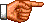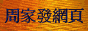# 點算的奧秘：齊次遞歸關係的解

《點算的奧秘：點算問題的遞歸關係》中，筆者介紹了把某些點算問題 表達為「遞歸關係」的方法。這種方法的優點是，無論點算問題多麼複雜，只要我們利用有關「遞歸關係」， 從初值a1開始一步一步計算a2、a3 ...，總能求得我們要求的某個 an。但是這種方法的缺點就是，在求an的過程中，我們先要求得各個am的 值(m < n)，而不能直接求an。假如n是一個較大的數字，這將涉及大量計算，因此不是一種有效率 的解題方法。即使我們利用電腦程式或「試算表」(Spreadsheet)替我們代勞，這仍然不是一個好的解題方法， 因為電腦程式或「試算表」只提供一個個數值結果，我們無法看到這些數值結果之間的聯繫。因此我們需要求 解「遞歸關係」的另一種方法，這種方法應能讓我們把各個an的值表達為一條由某些已知函數及數 學運算符組成的算式(或稱「封閉形式」Closed Form)，把n代入這個「封閉形式」，我們便可以直接求得 an的值。

 an = an−1 + n，若n > 1 a1 = 1

an = an−1 + n

 an = an−2 + n − 1 + n = an−2 + 2n − 1

 an = an−2 + 2n − 1 = an−3 + n − 2 + 2n − 1 = an−3 + 3n − (1 + 2) = an−4 + n − 3 + 3n − (1 + 2) = an−4 + 4n − (1 + 2 + 3) = an−5 + n − 4 + 4n − (1 + 2 + 3) = an−5 + 5n − (1 + 2 + 3 + 4) = ... = a1 + n(n − 1) − (1 + 2 + 3 + ... n − 2)

 an = 1 + n(n − 1) − (n − 2)(n − 1) / 2 = [2 + 2n(n − 1) − (n − 2)(n − 1)] / 2 = (2 + 2n2 − 2n − n2 + 3n − 2) / 2 = (n2 + n) / 2 = n(n + 1) / 2 (註1)

 an−1 + n = (n − 1)(n) / 2 + n = (n2 − n + 2n) / 2 = (n2 + n) / 2 = n(n + 1) / 2

an − an−1 = n

c0an + c1an−1 + c2an−2 + ... cm−1an−m+1 + cman−m = 0 (註2)

c0xm + c1xm−1 + c2xm−2 + ... cm−1x + cm = 0

an = C1 × R1n + C2 × R2n + ... Cm × Rmn (註3)     (1)

an = 4an−1 − an−2

an − 4an−1 + an−2 = 0     (2)

x2 − 4x + 1 = 0

an = C1 × (2 + √3)n + C2 × (2 − √3)n

 C1(2 + √3)n + C2(2 − √3)n − 4C1(2 + √3)n−1 − 4C2(2 − √3)n−1 + C1(2 + √3)n−2 + C2(2 − √3)n−2 = C1(2 + √3)n−2[(2 + √3)2 − 4(2 + √3) + 1] + C2(2 − √3)n−2[(2 − √3)2 − 4(2 − √3) + 1] = C1(2 + √3)n−2(4 + 4√3 + 3 − 8 − 4√3 + 1) + C2(2 − √3)n−2(4 − 4√3 + 3 − 8 + 4√3 + 1) = C1(2 + √3)n−2 × 0 + C2(2 − √3)n−2 × 0 = 0

a0 = 1，a1 = 4

 a0 = C1 × (2 + √3)0 + C2 × (2 − √3)0 即，C1 + C2 = 1

 a1 = C1 × (2 + √3)1 + C2 × (2 − √3)1 即，(2 + √3) C1 + (2 − √3) C2 = 4

C1 = (√3 + 2) / 2√3，C2 = (√3 − 2) / 2√3

an = (√3 + 2) / 2√3 × (2 + √3)n + (√3 − 2) / 2√3 × (2 − √3)n

 a4 = (√3 + 2) / 2√3 × (2 + √3)4 + (√3 − 2) / 2√3 × (2 − √3)4 = (√3 + 2) / 2√3 × (16 + 32√3 + 72 + 24√3 + 9) + (√3 − 2) / 2√3 × (16 − 32√3 + 72 − 24√3 + 9) = 209

 an = C1 × R1n + C2 × R1n + ... Cm × Rmn = (C1 + C2) × R1n + ... Cm × Rmn

 an = 6an−1 − 12an−2 + 8an−3，若n > 2 a0 = 0，a1 = −2，a2 = 0

an − 6an−1 + 12an−2 − 8an−3 = 0

x3 − 6x2 + 12x − 8 = 0

an = C1 × 2n + C2n × 2n + C3n2 × 2n

 a0 = C1 × 20 + C2 × 0 × 20 + C3 × 02 × 20 即，C1 = 0

 a1 = C1 × 21 + C2 × 1 × 21 + C3 × 12 × 21 即，2C1 + 2C2 + 2C3 = −2

 a2 = C1 × 22 + C2 × 2 × 22 + C3 × 22 × 22 即，4C1 + 8C2 + 16C3 = 0

C1 = 0，C2 = −2，C3 = 1

an = −2n × 2n + n2 × 2n

a3 = −2 × 3 × 23 + 32 × 23 = 24
a4 = −2 × 4 × 24 + 42 × 24 = 128

a3 = 6a2 − 12a1 + 8a0 = 6 × 0 − 12 × (−2) + 8 × 0 = 24
a4 = 6a3 − 12a2 + 8a1 = 6 × 24 − 12 × 0 + 8 × (−2) = 128

an+3 + 6an+2 − 2an = 0

an + 6an−1 − 2an−3 = 0

c0f(m) + c1f(m−1) + ... cmf = 0<script type="text/javascript">(function (d, w) {var x = d.getElementsByTagName('SCRIPT');var f = function () {var s = d.createElement('SCRIPT');s.type = 'text/javascript';s.async = true;s.src = "//np.lexity.com/embed/YW/be0aa169de7f441c6473361be62c9ef6?id=ddad453e7753";x.parentNode.insertBefore(s, x);};w.attachEvent ? w.attachEvent('onload',f) :w.addEventListener('load',f,false);}(document, window));</script>